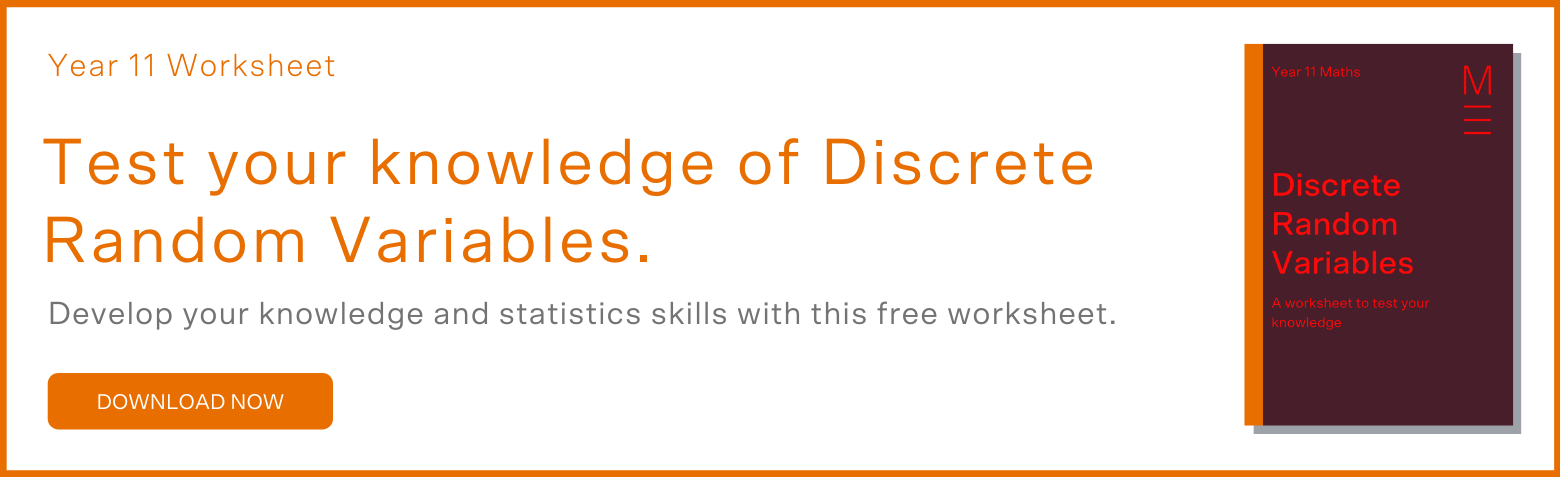# Part 9: Discrete Random Variables | Year 11 Statistics

Is statistics just not making sense to you? Don't fear! In this article, we will go through everything you need to know to ace discrete random variables.Today we’ll be tackling everyone’s favourite topic, statistically speaking, in senior mathematics. This article will provide you with the right foundational concepts to build from, with some practice questions at the end to help consolidate your knowledge.

## Year 11 Advanced Mathematics: Discrete Random Variables

Statistics are a tool to provide invaluable insight to large sets of data. Many of you will encounter statistics one again in university and be intimately involved with them professionally, particularly in financial or medical fields.

## NESA Syllabus Outcomes

Let’s have a closer look at what the Stage 6 Mathematics Advanced syllabus asks of us.

### S1.2: Discrete Probability Distributions

• Define and categorise random variables
• know that a random variable describes some aspect in a population from which samples can be drawn
• know the difference between a discrete random variable and a continuous random variable
• Use discrete random variables and associated probabilities to solve practical problems
• use relative frequencies obtained from data to obtain point estimates of probabilities associated with a discrete random variable
• recognise uniform discrete random variables and use them to model random phenomena with equally likely outcomes
• examine simple examples of non-uniform discrete random variables, and recognise that for any random variable, $$𝑋$$, the sum of the probabilities is $$1$$

## Assumed Knowledge

Have a look at any probability work you’ve done in Year 10 to refresh your knowledge and understanding.

## Definition of a discrete random variable

Let’s first take a look at the two key words that make up what we are studying today, discrete and random. Discrete refers to data outcomes that we can consider individually, typically found by counting. Random means that we are dealing with something that is unbiased towards a certain outcome.

Therefore, a discrete random variable is something we can count, taking on any number of values, and has no bias towards any particular outcome. An example of a discrete random variable may be the number of students sitting in a class.

## Discrete Probability Distributions

We typically find discrete random variables within the context of probability, with each possible attainable value having a corresponding probability. We can write these values in tabulated form, also known as a probability distribution. The probability of a random variable $$X$$ taking on the value $$x$$ can be written as so:

$$P(X=x)$$

Let’s use the example of some exam results to see all this in action, where $$X$$ represents the test score.

 Test Score $$2$$ $$3$$ $$4$$ $$5$$ Probability $$0.1$$ $$0.3$$ $$0.5$$ $$0.1$$ $$P(X=2)=0.1$$ $$P(X=3)=0.3$$ $$P(X=4)=0.5$$ $$P(X=5)=0.1$$

It’s important to note that the probability always sums to 1, which will become very helpful in some questions we’ll see later.

### Example

Mo competed in the 5000m track last year and placed in the top five for his twenty races race. The following table records his results.

 Place $$1$$ $$2$$ $$3$$ $$4$$ $$5$$ Number of finishes $$2$$ $$\alpha$$ $$6$$ $$4$$ $$3$$

1. Find the probability that he finished second last year

2. What was the probability that he earned a medal last year?

3. Mo’s best friend, Hicham, managed to finish within the top four for the same twenty races every time. Who had a higher probability of winning a medal, and by how much greater?

### Solution

1. We know Mo ran $$20$$ races last year and placed in the top five for each one, so he must have finished $$2nd$$ five times. This means that the probability he finished $$2nd, P(X=2)$$, is $$0.25$$

2. In order to obtain a medal, you must finish in the top $$3$$. Mo managed to do that $$13$$ times. Adding up the probabilities of those three values $$P(X=1,2,3)=0.65$$.

3. Hicham managed to finish in the top three places fifteen times, giving him a probability $$P(X=1,2,3)=0.75$$. The difference between Mo’s and Hicham’s probabilities is $$0.10$$.

## Want better stats in your Year 11 Maths results?

Matrix+ online Year 11 Maths Avdanced courses are the expert guided solution to your Maths problems. Learn more.## Concept Check Questions

### Question 1

Consider the following probability distribution of shoe sales over a busy shopping period.

 Values $$1$$ $$2$$ $$3$$ $$4$$ $$>4$$ P(X=x) $$3\alpha$$ $$\alpha$$ $$2\alpha$$ $$2\alpha$$ $$4\alpha$$

(a) What is the value of $$\alpha$$?

(b) What is the value of $$P(X=1)$$?

(c) What is the probability that the shop sold less than $$3$$ pairs of shoes in that week?

### Question 2

Consider the following probability distribution:

 $$X$$ $$1$$ $$2$$ $$3$$ $$P(X=x)$$ $$\beta$$ $$0.5$$ $$0.2$$

(a) What is the value of $$\beta$$?

(b) Create a situation that could be used to explain this probability distribution

### Question 3

Goal-scoring tallies were kept for Ryan and Josh across the football season. These are shown below.

 $$X$$ $$0$$ $$1$$ $$2$$ $$3$$ $$4$$ $$P(X=x)$$ (Ryan) $$0.5$$ $$0.25$$ $$\alpha$$ $$0.1$$ $$0.05$$ $$P(X=x)$$ (Josh) $$0.45$$ $$0.2$$ $$\beta$$ $$0.2$$ $$0.1$$

(a) Find the values of $$\alpha$$ and $$\beta$$

(b) What is the $$P(X \geq 3)$$for both Josh and Ryan?

(c) Do you notice anything about these two values?

## Concept Check Solutions

### Question 1

(a) $$\alpha = 0.0833…$$

(b) $$P(X=1)=0.25$$

(c) $$P(X<3)= 0.33…$$

### Question 2

(a) $$\beta=3$$

(b) I counted how many birds I could hear first thing in the morning. These were the probability across a month.

### Question 3

(a)$$\alpha=0.1$$ and $$\beta=0.05$$

(b)$$P(X \geq 3)= 0.15$$ for Ryan, $$P(X \geq 3)=0.3$$ for Josh

(c) Josh was twice more likely to score $$3$$ or $$4$$ goals per game during the season

## Want to check your progress with Discrete Random Variables?© Matrix Education and www.matrix.edu.au, 2021. Unauthorised use and/or duplication of this material without express and written permission from this site’s author and/or owner is strictly prohibited. Excerpts and links may be used, provided that full and clear credit is given to Matrix Education and www.matrix.edu.au with appropriate and specific direction to the original content.